### IMO Shortlist 1997 problem 9

Kvaliteta:
Avg: 3,0
Težina:
Avg: 7,0
Let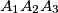$A_1A_2A_3$ be a non-isosceles triangle with incenter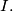$I.$ Let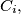$C_i,$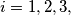$i = 1, 2, 3,$ be the smaller circle through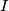$I$ tangent to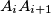$A_iA_{i+1}$ and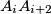$A_iA_{i+2}$ (the addition of indices being mod 3). Let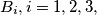$B_i, i = 1, 2, 3,$ be the second point of intersection of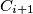$C_{i+1}$ and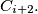$C_{i+2}.$ Prove that the circumcentres of the triangles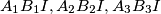$A_1 B_1I,A_2B_2I,A_3B_3I$ are collinear.
Izvor: Međunarodna matematička olimpijada, shortlist 1997